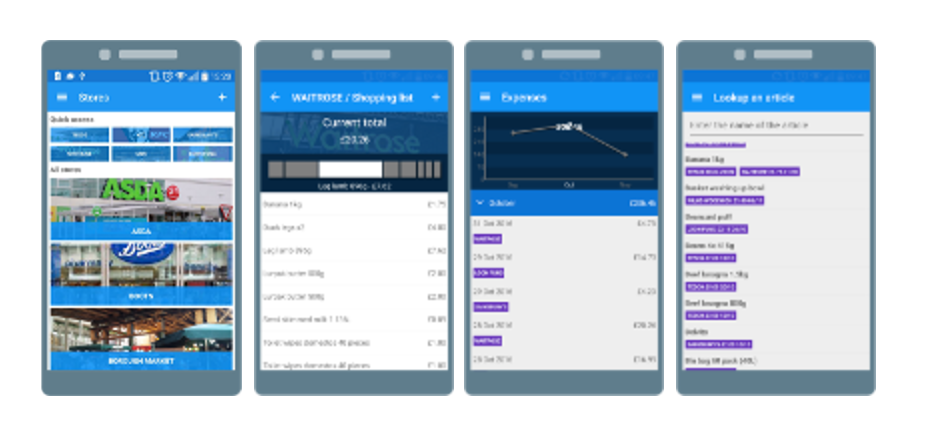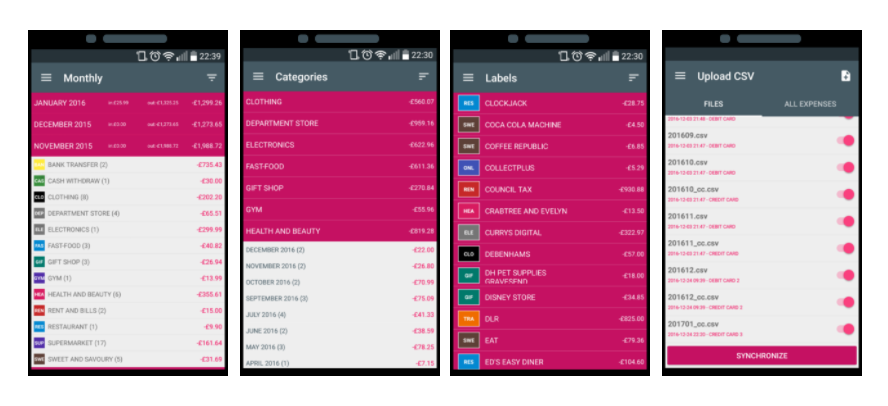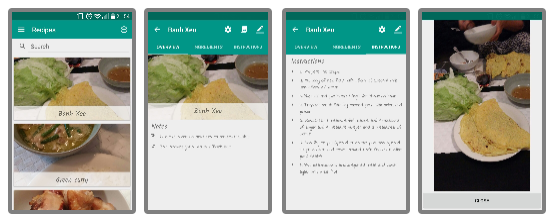### How to avoid input lost focus with ListModel WebSharper F#

20170202_listmodel_websharper_lostfocus.md

# How to avoid input lost focus with ListModel WebSharper F#

Few months ago, I explained how ListModel worked. Today I would like to share a recurring issue that I used to have - lost of focus on input every time the ListModel values change. There’s an easy solution to that which I will demonstrate by first showing the initial code and explaining what is going on, why the focus is lost, then I will explain how we can work around it.

This post will be composed by two parts:

`````` 1. Why the inputs lose focus?
2. How to prevent it
``````

I thought about sharing this when I saw that someone else has had the same issue - http://try.websharper.com/cache/0000Bj.

## 1. Why the inputs lose focus?

The code is the following:

``````[<JavaScript>]
module Lensing =

let aliases =
ListModel.Create id [ "Bill"; "Joe" ]
aliases.LensInto id (fun a n -> n) aliasKey
let Main =
div [
aliases.View

aliases.View

]
|> Doc.RunById "main"
``````

If you try this, you will see that the list gets updated but the input focus is lost after each changes.

The problem comes from the fact that the form itself is observing the list changes.
If we look at how the form is rendered, it is rendered in the `View callback` therefore every time we change the ListModel the whole form is re-rendered and since the old `dom` is removed, we lose focus on the input.

``````aliases.View
|> Doc.BindSeqCached (fun (aliasKey: string) -> Doc.Input [] (lensIntoAlias aliasKey)) // <<= This input is re-rendered every time aliases ListModel changes
``````

So what can we do about it?

## 2. How to prevent it

### 2.1 Number of elements doesn’t change

The first problem is that the `Key` used for the lens is the value in that example. So let’s fix this by giving each alias a key by introducing a type `Alias`.

``````type Alias = { Key: int; Value: string }

let aliases =
ListModel.Create (fun a -> a.Key) [ { Key = 1; Value = "Bill" }; { Key = 2; Value = "Joe" } ]
aliases.LensInto (fun a -> a.Value) (fun a n -> { a with Value = n }) aliasKey

``````

If the number of elements doesn’t change, we actually don’t need to observe the list. We can take its initial value and render the form. Like that the Dom will not be deleted each time.

``````type Alias = { Key: int; Value: string }

let aliases =
ListModel.Create (fun a -> a.Key) [ { Key = 1; Value = "Bill" }; { Key = 2; Value = "Joe" } ]
aliases.LensInto (fun a -> a.Value) (fun a n -> { a with Value = n }) aliasKey

let Main =
div
[
aliases.Value
|> Seq.map (fun al -> Doc.Input [] (lensIntoAlias al.Key))
|> Seq.cast
|> Doc.Concat

aliases.View
|> Doc.BindSeqCached (fun al -> div [ text al.Value ])
]
|> Doc.RunById "main"
``````

### 2.2 Number of elements needs to change

If we need to observe the list changes, observe when elements are added or removed, the form will have to be re-rendered and we will have to lose focus.
To work around that, we can use a `Snapshot` combined with a Update button.

``````View.SnapshotOn aliases.Value trigger.View
``````

`Snapshot` are used with a combined `Var`, I call it a `trigger`. It is just a `Var<unit>` which, when set, will trigger the refresh of the view.

So here’s how we use it:

``````let trigger =
Var.Create ()

let Main =
div
[
Doc.Button
[ attr.style "display: block" ]
(fun() ->
aliases.Add({ Key = aliases.Length + 1; Value = "New" })
// trigger update here
trigger.Value <- ())

aliases.View
|> View.SnapshotOn aliases.Value trigger.View
|> Doc.BindSeqCached (fun al -> Doc.Input [] (lensIntoAlias al.Key))

aliases.View
|> Doc.BindSeqCached (fun al -> div [ text al.Value ])
]
|> Doc.RunById "main"
``````

So when we add a new alias, we trigger an update of the form. This makes the form only render when the user click on Add. And as you can see, it's working fine now!

# Correction: Use Doc.BindSeqCachedViewBy

As Loïc pointed out:

You should take a look at Doc.BindSeqCachedViewBy. It does exactly what you need here: the rendered Docs are cached according to a key function, and the value is passed as a view to the renderer, so that the rendered content stays in place and only the moving parts vary.

`Doc.BindSeqCachedViewBy` does exactly what was needed without having to implement our little trick.
Here the code sample:

``````[<JavaScript>]
module Lensing =
type Alias = { Key: int; Value: string }

let aliases =
ListModel.Create (fun a -> a.Key) [ { Key = 1; Value = "Bill" }; { Key = 2; Value = "Joe" } ]
aliases.LensInto (fun a -> a.Value) (fun a n -> { a with Value = n }) aliasKey

let Main =
div
[
Doc.Button
[ attr.style "display: block" ]
(fun() ->
aliases.Add({ Key = aliases.Length + 1; Value = "New" }))

// Thanks to BindSeqCachedViewBy, the input stays when al.Value changes.
aliases.View
|> Doc.BindSeqCachedViewBy (fun al -> al.Key) (fun key vAl ->
Doc.Input [] (lensIntoAlias key))

// Similarly here, the div stays and only the textView changes.
aliases.View
|> Doc.BindSeqCachedViewBy (fun al -> al.Key) (fun key vAl ->
div [ textView (vAl.Map (fun al -> al.Value)) ])
]
|> Doc.RunById "main"
``````

By using `BindSeqCachedViewBy`, we can dictate precisely which element needs to be updated. This will allow us to not rerender the elements but instead render specific elements which will remove the problem of lost input and at the same time will improve performance.

# Conclusion

Today we saw how we could work around the problem of losing focus in input when ListModle gets updated. Hope you liked this post, if you have any question, leave it here or hit me on Twitter @Kimserey_Lam. See you next time!

# Support me!

Support me by visting my website. Thank you!1.You should take a look at Doc.BindSeqCachedViewBy. It does exactly what you need here: the rendered Docs are cached according to a key function, and the value is passed as a view to the renderer, so that the rendered content stays in place and only the moving parts vary. Here is your example converted: http://try.websharper.com/snippet/0000CE

1.very nice! Thanks for the correction, I'll amend the blog post!